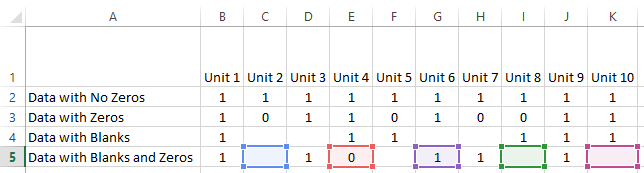# Challenge Answer – Negative AverageIf for Non-Continuous RangeIn our last Friday challenge:

Friday Challenge – AverageIf NOT Blank or NOT Zero with Negative Values for Non-Contiguous Ranges

I wanted to see how you could also take into account when a user puts in negative numbers into the Non-Contiguous Range as our previous formula would work only if the numbers were positive.

Here is the formula I came up with:

=SUM(C3,E3,G3,I3,K3)/(INDEX(FREQUENCY((C3,E3,G3,I3,K3),{-1,0}),3)+INDEX(FREQUENCY((C3,E3,G3,I3,K3),{-1,0}),1))

Chad R submitted this similar but BETTER response.

=SUM(C3,E3,G3,I3,K3)/INDEX(FREQUENCY((C3,E3,G3,I3,K3),0)+FREQUENCY((C3,E3,G3,I3,K3),-1),1)

Laura submitted this EASIER response:

=AVERAGEIFS(B2:K2,\$B\$3:\$K\$3,”X”,B2:K2,”<>0″)

Note: this formula needs a helper row of X’s inserted in Row 3 in order to tell Excel the non-contiguous range.

Leonid submitted the BEST one in my opinion:

{=SUM(C2,E2,G2,I2,K2)/COUNT((1/CHOOSE({1,2,3,4,5},C2,E2,G2,I2,K2)))}

Note: This is an Array formula.  Do not enter the  { and } brackets as Excel will enter these for you when you Press CTRL+SHIFT+ENTER when you are finished typing in the formula.

Check out Leonid’s solution.  It is way cool!

Here is the data set so you can play with the formulas presented above: